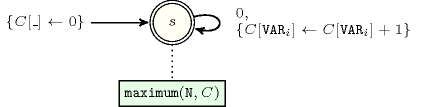## 5.244. max_nvalue

Origin

Derived from $\mathrm{𝚗𝚟𝚊𝚕𝚞𝚎}$.

Constraint

$\mathrm{𝚖𝚊𝚡}_\mathrm{𝚗𝚟𝚊𝚕𝚞𝚎}\left(\mathrm{𝙼𝙰𝚇},\mathrm{𝚅𝙰𝚁𝙸𝙰𝙱𝙻𝙴𝚂}\right)$

Arguments
 $\mathrm{𝙼𝙰𝚇}$ $\mathrm{𝚍𝚟𝚊𝚛}$ $\mathrm{𝚅𝙰𝚁𝙸𝙰𝙱𝙻𝙴𝚂}$ $\mathrm{𝚌𝚘𝚕𝚕𝚎𝚌𝚝𝚒𝚘𝚗}\left(\mathrm{𝚟𝚊𝚛}-\mathrm{𝚍𝚟𝚊𝚛}\right)$
Restrictions
 $\mathrm{𝙼𝙰𝚇}\ge 1$ $\mathrm{𝙼𝙰𝚇}\le |\mathrm{𝚅𝙰𝚁𝙸𝙰𝙱𝙻𝙴𝚂}|$ $\mathrm{𝚛𝚎𝚚𝚞𝚒𝚛𝚎𝚍}$$\left(\mathrm{𝚅𝙰𝚁𝙸𝙰𝙱𝙻𝙴𝚂},\mathrm{𝚟𝚊𝚛}\right)$
Purpose

$\mathrm{𝙼𝙰𝚇}$ is the maximum number of times that the same value is taken by the variables of the collection $\mathrm{𝚅𝙰𝚁𝙸𝙰𝙱𝙻𝙴𝚂}$.

Example
 $\left(3,〈9,1,7,1,1,6,7,7,4,9〉\right)$ $\left(1,〈9,1,7,3,2,6〉\right)$ $\left(6,〈5,5,5,5,5,5〉\right)$

In the first example, values $1,4,6,7,9$ are respectively used $3,1,1,3,2$ times. So the maximum number of time $\mathrm{𝙼𝙰𝚇}$ that a same value occurs is 3. Consequently the corresponding $\mathrm{𝚖𝚊𝚡}_\mathrm{𝚗𝚟𝚊𝚕𝚞𝚎}$ constraint holds.

Typical
 $\mathrm{𝙼𝙰𝚇}>1$ $\mathrm{𝙼𝙰𝚇}<|\mathrm{𝚅𝙰𝚁𝙸𝙰𝙱𝙻𝙴𝚂}|$ $|\mathrm{𝚅𝙰𝚁𝙸𝙰𝙱𝙻𝙴𝚂}|>1$ $\mathrm{𝚛𝚊𝚗𝚐𝚎}$$\left(\mathrm{𝚅𝙰𝚁𝙸𝙰𝙱𝙻𝙴𝚂}.\mathrm{𝚟𝚊𝚛}\right)>1$
Symmetries
• Items of $\mathrm{𝚅𝙰𝚁𝙸𝙰𝙱𝙻𝙴𝚂}$ are permutable.

• All occurrences of two distinct values of $\mathrm{𝚅𝙰𝚁𝙸𝙰𝙱𝙻𝙴𝚂}.\mathrm{𝚟𝚊𝚛}$ can be swapped; all occurrences of a value of $\mathrm{𝚅𝙰𝚁𝙸𝙰𝙱𝙻𝙴𝚂}.\mathrm{𝚟𝚊𝚛}$ can be renamed to any unused value.

Arg. properties

Functional dependency: $\mathrm{𝙼𝙰𝚇}$ determined by $\mathrm{𝚅𝙰𝚁𝙸𝙰𝙱𝙻𝙴𝚂}$.

Usage

This constraint may be used in order to replace a set of $\mathrm{𝚌𝚘𝚞𝚗𝚝}$ or $\mathrm{𝚊𝚖𝚘𝚗𝚐}$ constraints were one would have to generate explicitly one constraint for each potential value. Also useful for constraining the number of occurrences of the mostly used value without knowing this value in advance and without giving explicitly an upper limit on the number of occurrences of each value as it is done in the $\mathrm{𝚐𝚕𝚘𝚋𝚊𝚕}_\mathrm{𝚌𝚊𝚛𝚍𝚒𝚗𝚊𝚕𝚒𝚝𝚢}$ constraint.

Reformulation

Assume that $\mathrm{𝚅𝙰𝚁𝙸𝙰𝙱𝙻𝙴𝚂}$ is not empty. Let $\alpha$ and $\beta$ respectively denote the smallest and largest possible values that can be assigned to the variables of the $\mathrm{𝚅𝙰𝚁𝙸𝙰𝙱𝙻𝙴𝚂}$ collection. Let the variables ${O}_{\alpha },{O}_{\alpha +1},\cdots ,{O}_{\beta }$ respectively correspond to the number of occurrences of values $\alpha ,\alpha +1,\cdots ,\beta$ within the variables of the $\mathrm{𝚅𝙰𝚁𝙸𝙰𝙱𝙻𝙴𝚂}$ collection. The $\mathrm{𝚖𝚊𝚡}_\mathrm{𝚗𝚟𝚊𝚕𝚞𝚎}$ constraint can be expressed as the conjunction of the following two constraints:

$\mathrm{𝚐𝚕𝚘𝚋𝚊𝚕}_\mathrm{𝚌𝚊𝚛𝚍𝚒𝚗𝚊𝚕𝚒𝚝𝚢}$ $\left(\mathrm{𝚅𝙰𝚁𝙸𝙰𝙱𝙻𝙴𝚂},$

$〈\mathrm{𝚟𝚊𝚕}-\alpha \mathrm{𝚗𝚘𝚌𝚌𝚞𝚛𝚛𝚎𝚗𝚌𝚎}-{O}_{\alpha },$

$\mathrm{𝚟𝚊𝚕}-\alpha +1\mathrm{𝚗𝚘𝚌𝚌𝚞𝚛𝚛𝚎𝚗𝚌𝚎}-{O}_{\alpha +1},$

$\cdots$

$\mathrm{𝚟𝚊𝚕}-\beta \mathrm{𝚗𝚘𝚌𝚌𝚞𝚛𝚛𝚎𝚗𝚌𝚎}-{O}_{\beta }〉\right)$,

$\mathrm{𝚖𝚊𝚡𝚒𝚖𝚞𝚖}$$\left(\mathrm{𝙼𝙰𝚇},〈{O}_{\alpha },{O}_{\alpha +1},\cdots ,{O}_{\beta }〉\right)$.

Counting
 Length ($n$) 2 3 4 5 6 7 8 Solutions 9 64 625 7776 117649 2097152 43046721

Number of solutions for $\mathrm{𝚖𝚊𝚡}_\mathrm{𝚗𝚟𝚊𝚕𝚞𝚎}$: domains $0..n$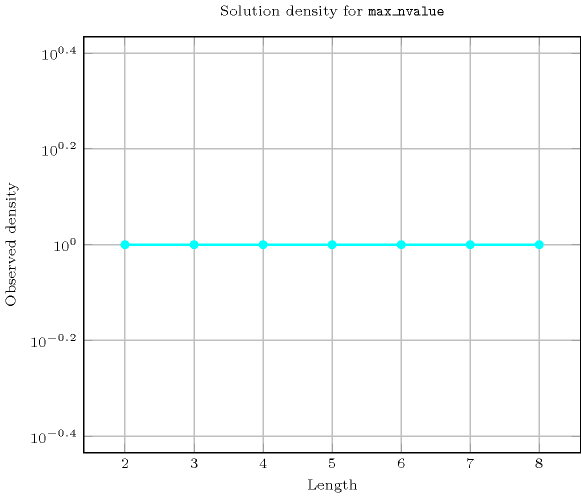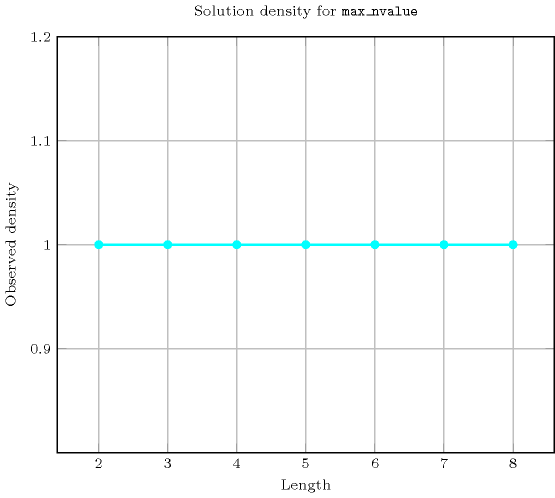Length ($n$)2345678
Total9646257776117649209715243046721
 Parameter value

1624120720504040320362880
2336420540078750130536024449040
3-48015002982064680015382080
4--51503780960402577960
5---62528232258048
6----739216128
7-----8576
8------9

Solution count for $\mathrm{𝚖𝚊𝚡}_\mathrm{𝚗𝚟𝚊𝚕𝚞𝚎}$: domains $0..n$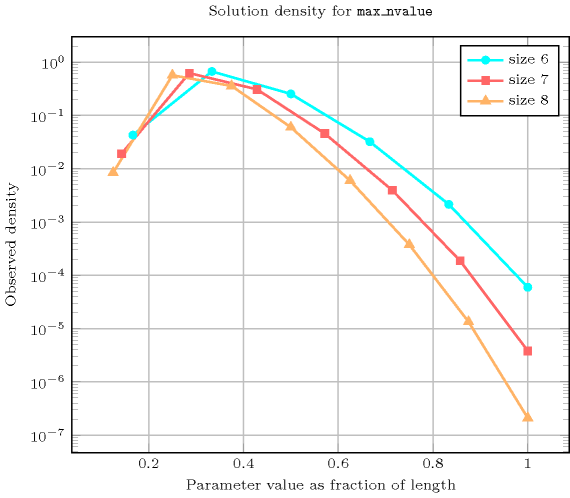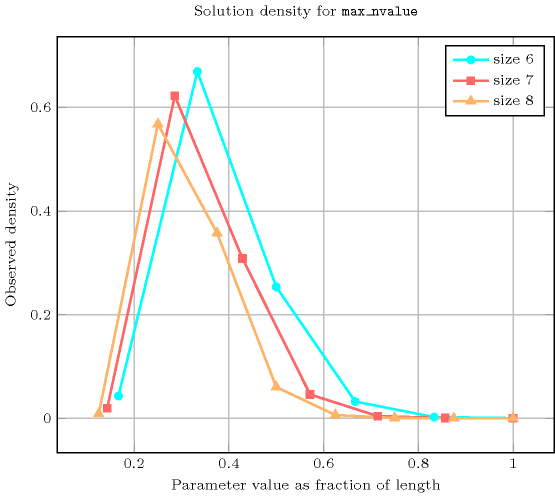Keywords
Arc input(s)

$\mathrm{𝚅𝙰𝚁𝙸𝙰𝙱𝙻𝙴𝚂}$

Arc generator
$\mathrm{𝐶𝐿𝐼𝑄𝑈𝐸}$$↦\mathrm{𝚌𝚘𝚕𝚕𝚎𝚌𝚝𝚒𝚘𝚗}\left(\mathrm{𝚟𝚊𝚛𝚒𝚊𝚋𝚕𝚎𝚜}\mathtt{1},\mathrm{𝚟𝚊𝚛𝚒𝚊𝚋𝚕𝚎𝚜}\mathtt{2}\right)$

Arc arity
Arc constraint(s)
$\mathrm{𝚟𝚊𝚛𝚒𝚊𝚋𝚕𝚎𝚜}\mathtt{1}.\mathrm{𝚟𝚊𝚛}=\mathrm{𝚟𝚊𝚛𝚒𝚊𝚋𝚕𝚎𝚜}\mathtt{2}.\mathrm{𝚟𝚊𝚛}$
Graph property(ies)
$\mathrm{𝐌𝐀𝐗}_\mathrm{𝐍𝐒𝐂𝐂}$$=\mathrm{𝙼𝙰𝚇}$

Graph model

Because of the arc constraint, each strongly connected component of the final graph corresponds to a distinct value that is assigned to a subset of variables of the $\mathrm{𝚅𝙰𝚁𝙸𝙰𝙱𝙻𝙴𝚂}$ collection. Therefore the number of vertices of the largest strongly connected component is equal to the mostly used value.

Parts (A) and (B) of Figure 5.244.1 respectively show the initial and final graph associated with the first example of the Example slot. Since we use the $\mathrm{𝐌𝐀𝐗}_\mathrm{𝐍𝐒𝐂𝐂}$ graph property, we show the largest strongly connected component of the final graph.

##### Figure 5.244.1. Initial and final graph of the $\mathrm{𝚖𝚊𝚡}_\mathrm{𝚗𝚟𝚊𝚕𝚞𝚎}$ constraint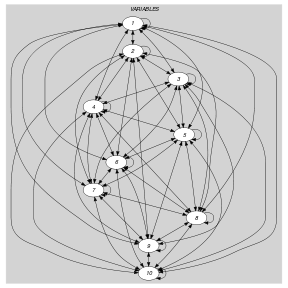(a)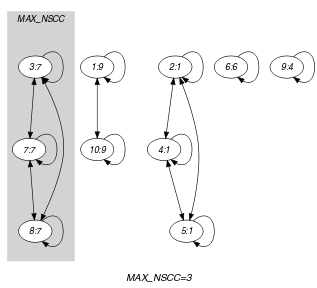(b)
Automaton

Figure 5.244.2 depicts the automaton associated with the $\mathrm{𝚖𝚊𝚡}_\mathrm{𝚗𝚟𝚊𝚕𝚞𝚎}$ constraint. To each item of the collection $\mathrm{𝚅𝙰𝚁𝙸𝙰𝙱𝙻𝙴𝚂}$ corresponds a signature variable ${S}_{i}$ that is equal to 0.

##### Figure 5.244.2. Automaton of the $\mathrm{𝚖𝚊𝚡}_\mathrm{𝚗𝚟𝚊𝚕𝚞𝚎}$ constraint